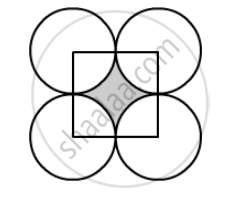Advertisement Remove all ads

# The Diameter of a Coin is 1 Cm (In the Following Figure). If Four Such Coins Be Placed on a Table So that the Rim of Each Touches that of the Other Two, Find the Area of the Shad - Mathematics

Sum

The diameter of a coin is 1 cm (in the following figure). If four such coins be placed on a table so that the rim of each touches that of the other two, find the area of the shaded region (Take π = 3.1416).Advertisement Remove all ads

#### Solution

Look at the figure carefully shaded region is bounded between four sectors of the circle with same radius and a square of side 1 cm.

Therefore, the area of the shaded region is nothing but the difference the area of the square and area of one circle.

"∴ Area of the shaded region=Area of square-Area of a circle"

"∴ Area of the shaded region"=1^2-pi (0.5^2)

"∴ Area of the shaded region"=1-0.25pi

Substituting pi=3.1416 we get,

"∴ Area of the shaded region"=1-3.1416xx0.25

"∴ Area of the shaded region"=1-0.7854

"∴ Area of the shaded region"=0.2146

Therefore, area of the shaded region is 0.2146cm^2

Is there an error in this question or solution?
Advertisement Remove all ads

#### APPEARS IN

RD Sharma Class 10 Maths
Chapter 13 Areas Related to Circles
Exercise 13.4 | Q 33 | Page 61
Advertisement Remove all ads

#### Video TutorialsVIEW ALL 

Advertisement Remove all ads
Share
Notifications

View all notifications

Forgot password?
Course